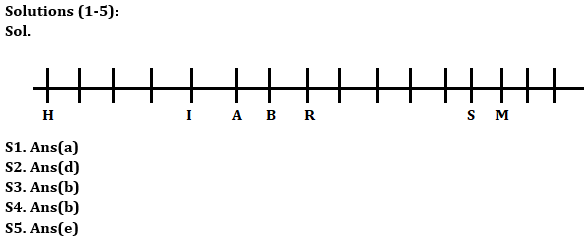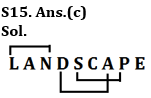Latest Banking jobs   »

# Reasoning Quiz For Bank of Baroda SO 2023 -10th May

Directions (1-5): Study the information carefully and answer the questions given below.
An uncertain number of persons sit in a row in such a way that all of them faces towards north direction. Four Persons sit between H and A. R sits second to the right of A. I sits third to the left of R. As many persons sit between S and B as many between B and H. Four persons sit between R and S. H is not immediate neighbor of B. M sits seventh to the right of B and third from one of the extreme ends of row. I sits fifth from the one of the extreme end of the row. Not more than three persons sit between I and H.

Q1. How many persons sit in the row?
(a) 16
(b) 17
(c) 15
(d) 14
(e) 18

Q2. Who among the following person sit second to the right of the one who sits eleventh from the right end of row?
(a) A
(b) B
(c) I
(d) R
(e) S

Q3. How many persons sit between I and S?
(a) Six
(b) Seven
(c) Eight
(d) Five
(e) Nine

Q4. If as many persons sit between A and T as many between T and M then how is T related to M?
(a) Third to the left
(b) Fourth to the left
(c) Fifth to the right
(d) Fourth to the right
(e) None of these

Q5. If Y sits exactly between H and I then how is B related to Y?
(a) Third to the right
(b) Fourth to the left
(c) Second to the right
(d) Immediate left
(e) None of these

Directions (6-10): Following questions are based on the five three-digit numbers given below.

645 826 721 395 548

Q6. What will be the difference when second digit of the 3rd lowest number is multiplied with the first digit of the highest number and first digit of the 2nd highest number is multiplied with the second digit of the lowest number?
(a) 21
(b) 30
(c) 31
(d)26
(e) None of these

Q7. If all the digits in each of the numbers are arranged in increasing order within the number, then, which of the following number will become the lowest in the new arrangement of numbers?
(a) 826
(b) 395
(c) 645
(d) 721
(e) 548

Q8. If one is added to the third digit of each of the numbers and one is subtracted to the second digit of each number then, how many numbers thus formed will be completely divisible by two in new arrangement?
(a) Three
(b) One
(c) None
(d) Two
(e) Four

Q9. If all the numbers are arranged in decreasing order from right to left then, which of the following will be the sum of all the three digits of the number which is 4th from the left in the new arrangement?
(a) 10
(b) 15
(c) 16
(d) 17
(e) None of these

Q10. If the positions of the first and the third digits of each of the numbers are interchanged then, how many even numbers will be formed?
(a) One
(b) Two
(c) Three
(d) None
(e) Four

Q11. If all the vowels of the word ‘TREATMENT’ are replaced by its succeeding letter according to the English alphabet and all the consonant are replaced with its previous letter according to the English alphabet and then all the letters are arranged in the alphabetical order from left, then how many letters are there between the third letter from the left and fourth letter from the right in the English alphabetic series?
(a) 6
(b) 12
(c) 9
(d) 10
(e) 11

Q12. Which alphabet is 3rd from the left in the meaningful four-letter word formed from the 1st, 2nd, 5th and 8th letter of the word “JOURNALIST”? If more than one word is formed, then mark answer as X and no meaningful word is formed then mark answer as Z.
(a) O
(b) J
(c) Z
(d) I
(e) X

Q13. If in the number “364872159”, 2 is multiply by the digits which is less than 5 and 1 is subtracted to the digits which is greater than 4, then all digits are arranged in ascending order from left to right, then which of the following digit is exactly middle in the number?
(a) 7
(b) 6
(c) 5
(d) 4
(e) None of these

Q14 Which of the following elements should come in a place ‘?’ ?
DW2 GT4 JQ16 ?
(a) LO30
(b) NM8
(c) MO231
(d) MN256
(e) None of these

Q15. How many pairs of letters (both forward and backward direction) are there in the word “LANDSCAPE” each of which have as many letters between them as they have in the English alphabetical series?
(a) One
(b) More than Three
(c) Three
(d) Two
(e) None

SolutionsSolutions (6-10):
S6. Ans(c)
S7. Ans(d)
S8. Ans(a)
S9. Ans(a)
S10.Ans(b)

S11. Ans.(d)
Sol. Original Word- TREATMENT
After Applied given conditions- BFFLMQSSS
10 letters are in between F and Q.

S12. Ans.(d)
Sol. JOIN

S13. Ans.(b)
Sol. Original Number- 364872159
After applied given conditions-244566788

S14. Ans.(d)## FAQs

### What is the selection process of Bank of Baroda SO 2023?

The selection process of Bank of Baroda SO consists of Online exam and Interview

#### Congratulations!Union Budget 2023-24: Free PDF